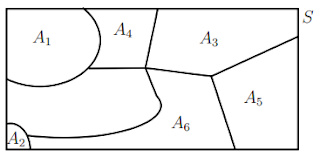# Total Probability and Baye's theorem

## Total Probability:

A sample space is divided into several non overlapping zones, and the sum of all these zones is equal to the sample space itself.For example a person takes different conveyance while going to his office. Probabilities that he takes car, bus and scooter are 30%, 40% and 30% respectively.  Here sum of these probabilities must equal to 1.

Now consider an event B which tell us that the probability of his getting late to his office when he takes the above said transports.  say, 10%, 35%, 5%.By using total probability we can calculate the probability of his late arrival at office.
$P(B) = P({A_1}).P(B|{A_1})$ + $P({A_2}).P(B|{A_2})$ + ........+ $P({A_n}).P(B|{A_n})$

1. A person has undertaken a construction job. The probabilities are 0.65 that there will be strike, 0.8 that the job will be completed on time if there is no strike, 0.32 that the job will be completed on time.  If there is a strike.  Determine the probability that the job will be completed on time.
Assume A = There will be a strike
B = Construction Job will be completed on time
P(A) = 0.65 and $P({A_1})$ = 1 - 0.65 = 0.35
P(B|A) = 0.32 and $P(B|{A_1}) = 0.8$
$P(B) = P(A).P(B|A) + P({A_1}).P(B|{A_1})$
= $0.65 \times 0.32 + 0.35 \times 0.8 = 0.488$

## Baye's theorem:

Assume that in the above example, the work has completed on time.  Now What is the probability that there will be strike or what is the probability that there will be no strike? That means we have to calculate $P({A_K}|B)$
$P({A_K}|B) = \displaystyle\frac{{P({A_k} \cap B)}}{{P(B)}}$ = $\displaystyle\frac{{P({A_k}).P(B|{A_k})}}{{P({A_1}).P(B|{A_1}) + ..... + P({A_n}).P(B|{A_n})}}$

2. There are 3 bags each containing 5 white and 2 black balls, and 2 bags each containing 1 white ball and 4 black balls.  A black ball having been drawn, find the chance it came from the first group.
Probability that the chosen bag is from the first group = $P({A_1}) = \displaystyle\frac{3}{5}$
Probability that the chosen bag is from the Second group = $P({A_2}) = \displaystyle\frac{2}{5}$
Probability to draw a black ball from the first bag = $P(B|{A_1}) = \displaystyle\frac{2}{7}$
Probability to draw a black ball from the second bag = $P(B|{A_2}) = \displaystyle\frac{4}{5}$
Total Probability to draw a black ball = P(B) = ${P({A_1}).P(B|{A_1}) + P({A_2}).P(B|{A_2})}$ = $\displaystyle\frac{3}{5} \times \frac{2}{7} + \frac{2}{5} \times \frac{4}{5}$
The probability that it is from the first group given that it is a black ball = $P({A_1}|B) = \displaystyle\frac{{P({A_1} \cap B)}}{{P(B)}}$ = $\displaystyle\frac{{P({A_1}).P(B|{A_1})}}{{P({A_1}).P(B|{A_1}) + P({A_2}).P(B|{A_2})}}$ = $\displaystyle\frac{{\displaystyle\frac{3}{5} \times \frac{2}{7}}}{{\displaystyle\frac{3}{5} \times \frac{2}{7} + \frac{2}{5} \times \frac{4}{5}}} = \frac{{15}}{{43}}$

3. Suppose, three bulb manufacturing plants A, B, C operates in a place. Let the production by these plants are 60%, 30%, 10% respectively.  Also defective bulbs produced by these plants are 5%, 10%, 10% respectively.  Of the total bulbs, one bulb was selected randomly and the found to be defective.  What is the probability that this bulb was manufactured by Plant A?
We are asked to find P(A | Def)
From conditional probability we know that P(A | Def) = $$\dfrac{{P(A \cap Def)}}{{P(Def)}} = \dfrac{{P(A) \times P(Def|A)}}{{P(Def)}}$$
P(Def) = P(A).P(Def | A) + P(B).P(Def | B) + P(C).P(Def | C)
So P(Def) = 60%.5% + 30%.10% + 10%.10% = 7% (of the total bulbs, 7% are defective)
P(A | Def) = $$\dfrac{{P(A \cap Def)}}{{P(Def)}}$$ = $$\dfrac{{P(A) \times P(Def|A)}}{{P(Def)}}$$ = $$\dfrac{{60\% \times 5\% }}{{7\% }} = \dfrac{3}{7}$$

4. A man is known to speak truth 3 out of 4 times. He throws die and reports that it is a 6. The probability that it is actually a 6 is
Sol: If 6 actually appeared, he can report it with the probability of 3/4.  If 6 has not appeared, still he can report it wrongly with the probability of 1/4
So the probability that it is actually a 6 = (Probability to appear 6 x His truthfulness to report + Probability to appear any other number x His lieing probability ) = $\displaystyle\displaystyle\frac{1}{6} \times \displaystyle\frac{3}{4} + \displaystyle\frac{5}{6} \times \displaystyle\frac{1}{4} = \displaystyle\frac{1}{3}$
The probability that it is actually 6 = $\displaystyle\frac{{{\rm\text{Probability that he reports 6}}}}{{{\rm\text{Total probability to appear 6}}}}$ = $\displaystyle\frac{{\displaystyle\frac{3}{4} \times \displaystyle\frac{1}{6}}}{{\displaystyle\frac{3}{4} \times \displaystyle\frac{1}{6} + \displaystyle\frac{1}{4} \times \displaystyle\frac{5}{6}}} = \displaystyle\frac{3}{8}$